# huangx607087学习分组密码的笔记3

## 3.DES,AES的加密模式

### 0o01 电子密码本模式 ECB

ECB模式是最简单的一种模式，实际上就是直接加密和直接解密，不需要其他的任何成分，仅需要明文$x$和密钥$k$，就可以获得密文$y$，当然，用$y$和$k$也可以通过解密得到$x$。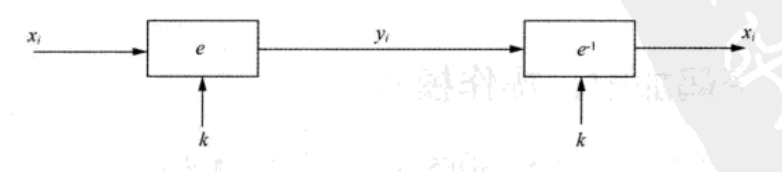ECB模式的优点就是如果在加密时，某个分组的内容出现了错误，后面的内容不会因为这个错误而受到影响，并且加密速度快、效率高、易操作。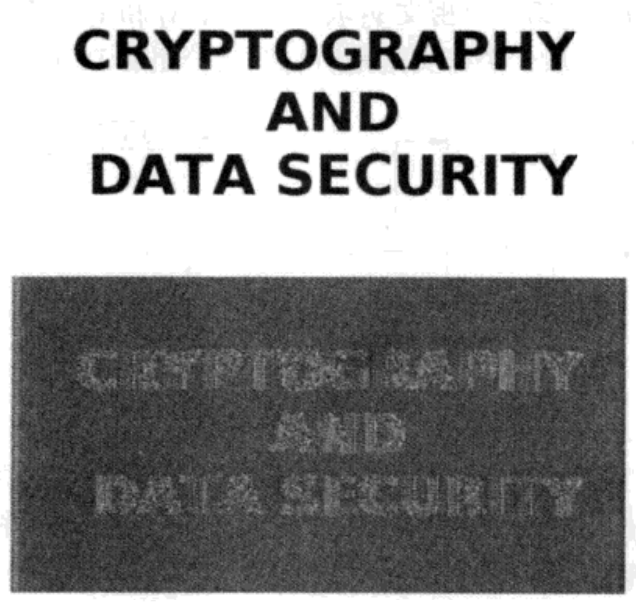### 0o02 密码分组链接模式(CBC)

CBC模式跟ECB模式不同：首先，对于某一组的加密不仅依赖于这一组的内容，还依赖于前面所有的明文分组。并且会使用初始向量IV对加密进行随机化。设$\mathrm {IV}=y_0$，那么我们有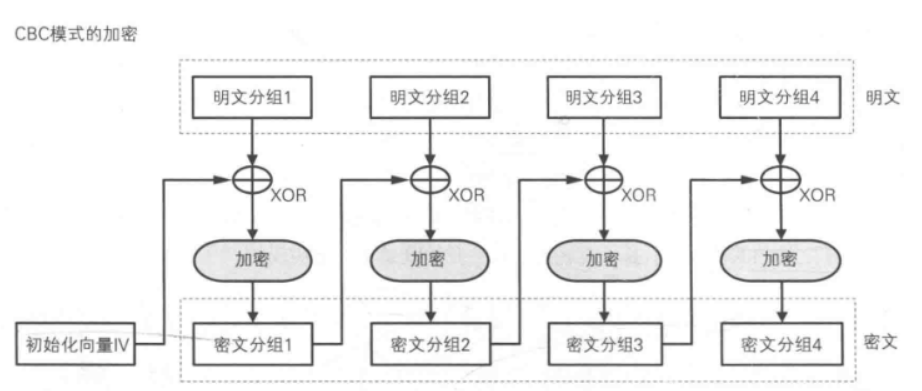### 0o03 输出反馈模式(OFB)

OFB模式是对初始化向量$iv$进行加密，然后每次对加密后的$iv$直接异或明文$x$，从而获得密文$y$的一种模式。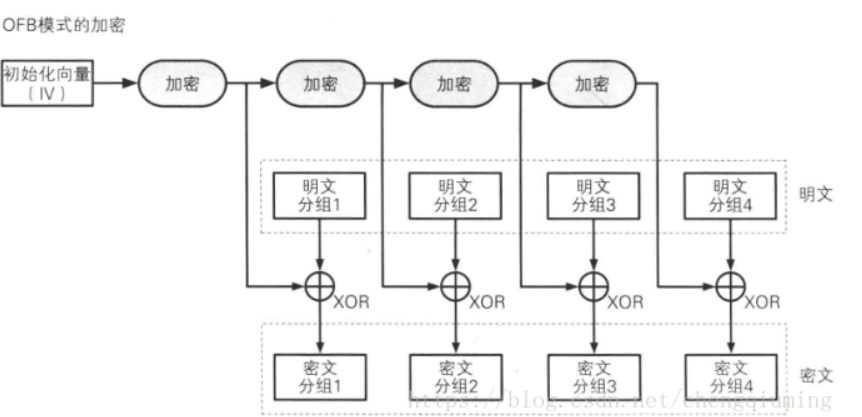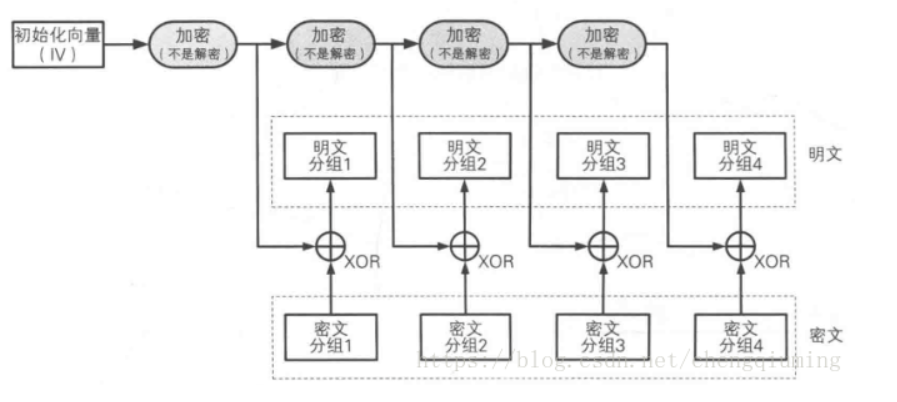### 0o04 密码反馈模式(CFB)

CFB，即密文反馈模式，仍然是对IV进行加密，然后与明文$x$进行异或得到密文$y$。然后$E(IV)$会继承后半部分内容作为新的前半部分，而新的后半部分则由密文的后半部分组成。如果是$16$位，替换$8$位。那么就是新的IV的前$8$位是旧的IV的后$8$位，然后新的IV的后$8$位是密文的后$8$位（当然也可以是前$8$位）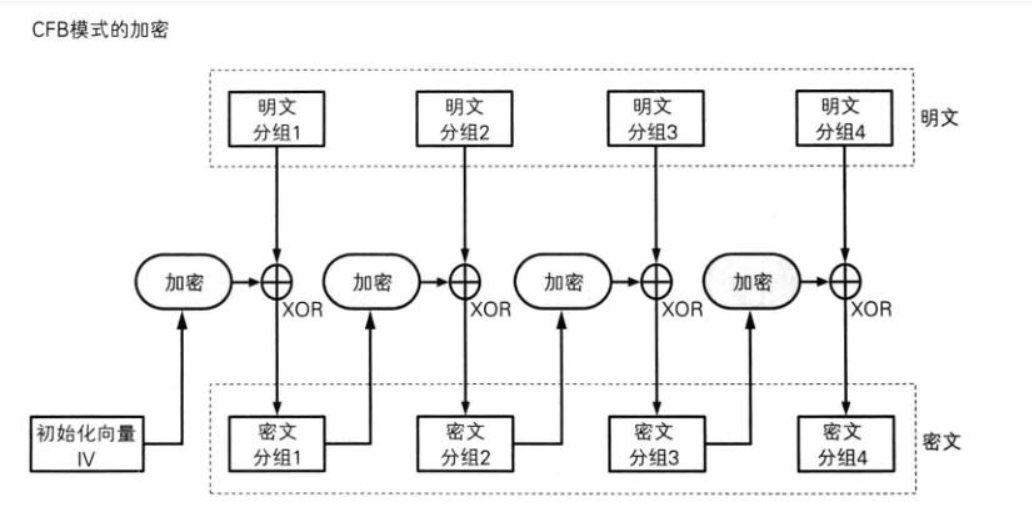### 0o05 计数器模式(CTR)

CTR模式这里有一个计数器。比如在$64$位的分组加密中，IV只提供$48$位，剩下的$16$位分配给计数器。例如：如果我们设$\text{IV = 86 43 23 6A 46 57H}$，那么一开始的$\text{IV=86 43 23 6A 46 57 00 00H}$，第二轮加密时用$\text{IV=86 43 23 6A 46 57 00 01H}$。每一次加密时，计数器会保持递增，但IV会一直不变。因此，十六位的计数器可以加密六万多组数据。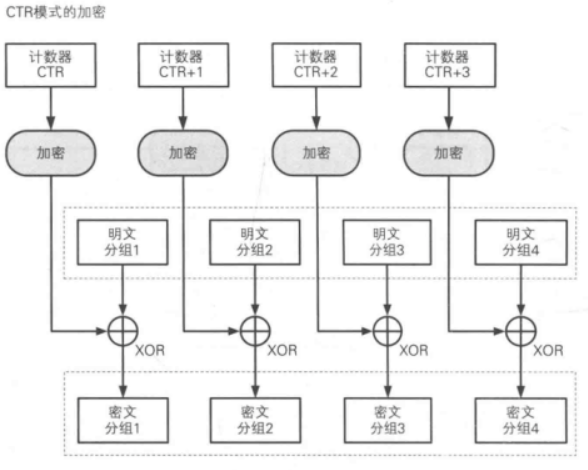### 0o06 GF域计数器模式(GCM)

GCM模式，使用伽罗瓦域乘法，这里是$GF(2^{128})$。在计算每个明文时都会得到一个中间认证参数$g$，$g_{i}$为当前密文$y_i$异或后，与一个常数$h$相乘。这里使用不可约多项式$x^{128}+x^7+x^2+x+1$。因为这里面只有一个乘法，因此GCM的计算开销不会很大。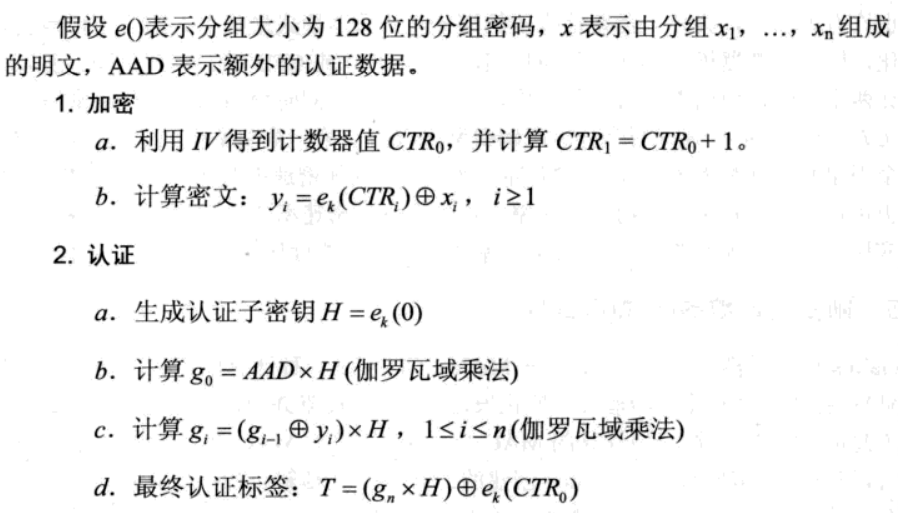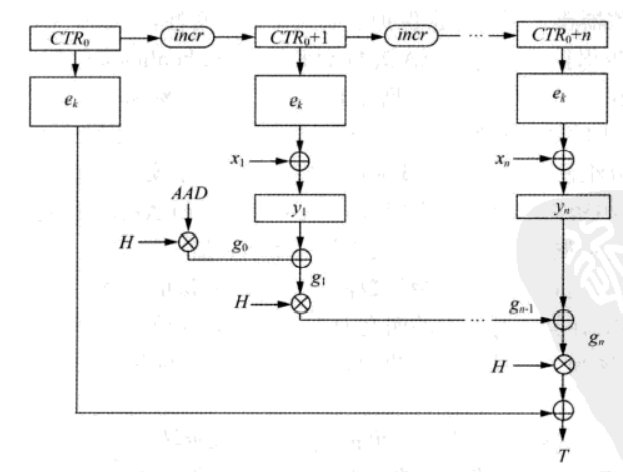目录